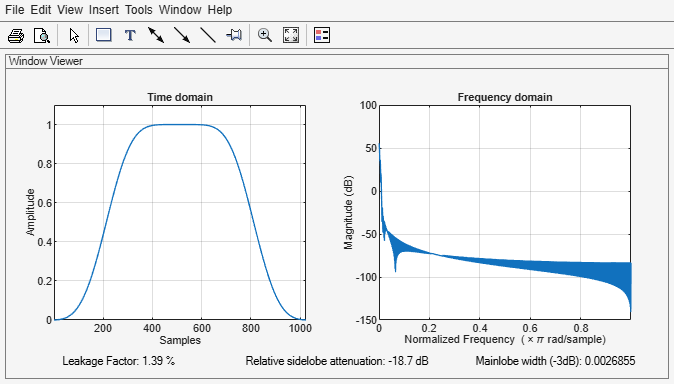# kbdwin

Kaiser-Bessel-derived window

## Syntax

``wdw = kbdwin(N)``
``wdw = kbdwin(N,Beta)``

## Description

example

````wdw = kbdwin(N)` returns an `N`-point Kaiser-Bessel-derived (KBD) window.```

example

````wdw = kbdwin(N,Beta)` specifies the tuning parameter, `Beta`.```

## Examples

collapse all

Create a 1024-point Kaiser-Bessel-derived (KBD) window. Visualize the KBD window in the time and frequency domains using `wvtool`.

```wdw = kbdwin(1024); wvtool(wdw)```Create three 512-point KBD windows, with `Beta` set to 1, 10, and 100. Display the windows for comparison using `wvtool`.

```N = 512; beta1 = kbdwin(N,1); beta10 = kbdwin(N,10); beta100 = kbdwin(N,100); wvtool(beta1,beta10,beta100)```## Input Arguments

collapse all

Number of points in the KBD window, specified as an even positive integer scalar.

Data Types: `single` | `double`

Tuning parameter, specified as a nonnegative real scalar. If unspecified, `Beta` defaults to `5`.

Data Types: `single` | `double`

## Output Arguments

collapse all

Kaiser-Bessel-derived window, returned as an `N`-point column vector.

## Algorithms

The coefficients of a Kaiser-Bessel-derived window are computed using the equation:

where w is a Kaiser window designed using the `kaiser` function:

`w = kaiser(N/2+1,Beta*pi)`
where `N` is the number of points in the KBD window and `Beta` is the tuning parameter.

 Bosi, Marina, and Richard E. Goldberg. Introduction to Digital Audio Coding and Standards. Dordrecht: Kluwer, 2003.

## SupportGet trial now